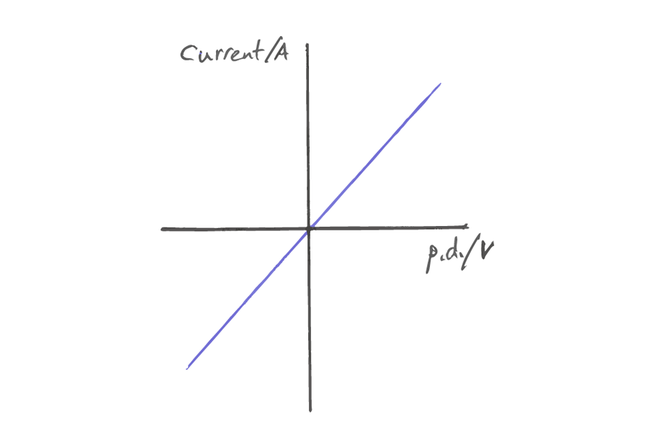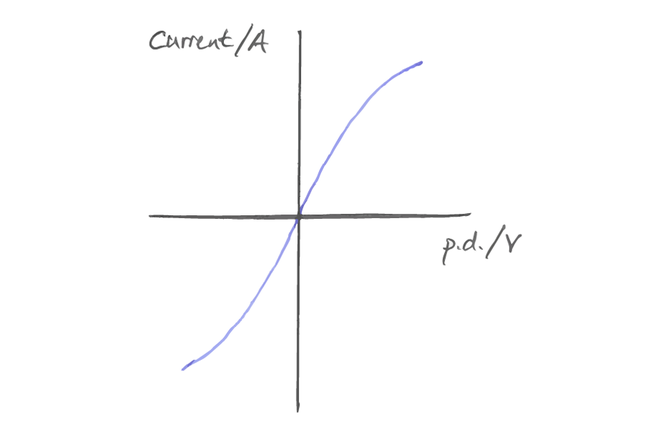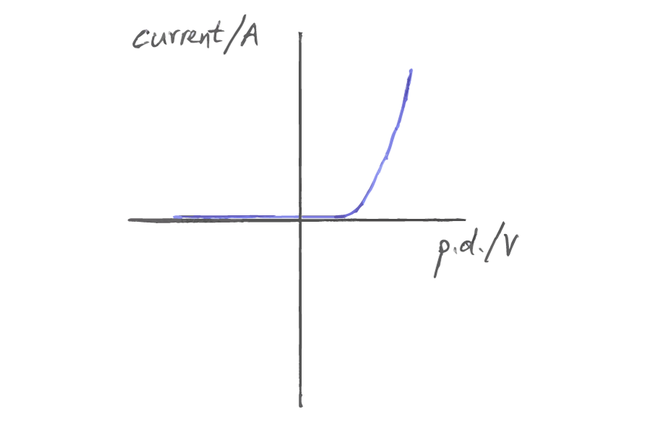## Want to keep learning?

This content is taken from the National STEM Learning Centre's online course, Teaching Practical Science: Physics. Join the course to learn more.
3.9

# A primer in I-V characteristics

In the next step, we look at practical work exploring current-voltage characteristics of some components. Here we provide a quick primer if you are not familiar with this topic.

The current, I, through a component is determined by the voltage applied across the components, V, and the resistance of the component, R.

Ohm’s Law is commonly remembered as V = IR, but since the current is dependent variable, it should really be described as I = V / R.

To reverse the current flow in the circuit, we simply swap the wires on the power supply terminals. This will give us negative readings of both voltage and current.

## Resistors

For resistors at low voltage, the relationship between current and voltage is linear: as you double the applied voltage, the current flowing through the circuit will double. So for voltages that we would use in the classroom, we would expect a straight line graph for a plot of I vs. V.

### Potential pit-falls

The resistor used is a high value (i.e. in the kilo-ohm region) and therefore the current will be low. This is OK if a digital multi-meter (DMM) is used and set to reads in mA. Typical values to use with a battery supply are resistors of 10 - 100 Ohms.Example I-V graph shape for resistor

## Bulbs

For a filament bulb, the relationship is non-linear. As we increase the voltage across the bulb, the flow of current causes the filament to heat up due to the vibration of the ions. This vibration also impedes the flow of the electrons. So a doubling of the voltage will not cause a doubling in the current.

### Potential pit-falls

If the graph does not curve, this could be because of a mismatch between the bulb rating and the power supply. For example, a 12V bulb with a 6V power supply may only show a linear relationship.Example I-V graph shape for filament bulb

## Diodes

A diode, whether it be a silicon diode or Light Emitting Diode (LED), is a semiconductor. Most diodes will only let current flow in one direction, and a threshold voltage must be reached before current flows. Once this voltage is reached, the current increases exponentially, and the component has extremely low resistance.

### Potential pit-falls

If a protective resistor is not used in series with the diode then this can cause the diode to break, and even explode. LEDs are often rated at around 20mA, so a sensible resistor value would be 100 ohms.Example I-V graph shape for diode